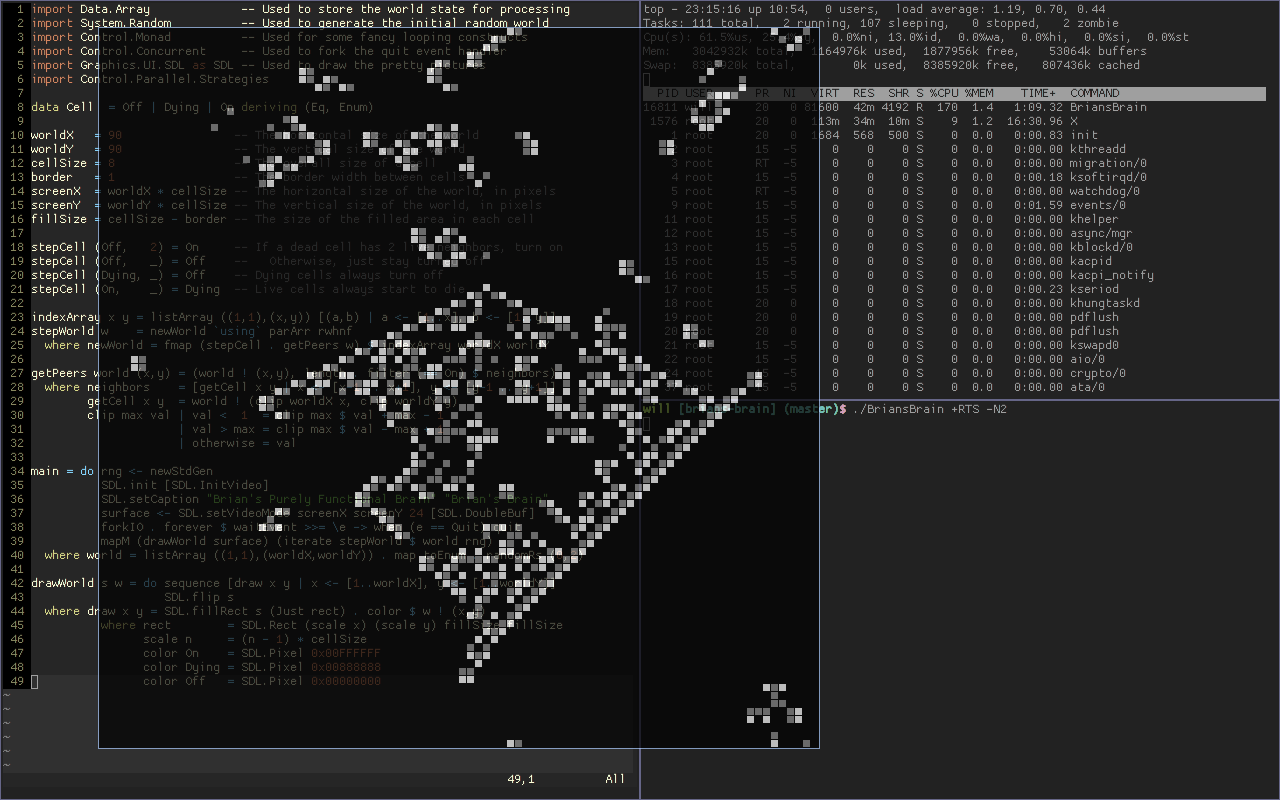← Will DonnellyBrian’s Brain

So about a week ago I came across an interesting post in which the author implemented the [Brian’s Brain](http://en.wikipedia.org/wiki/Brian’s_Brain) cellular automaton in 67 lines of Clojure. Not about to let my favorite language be outdone, I thought I’d see how well Haskell would do with the same task. Then I was kept horribly busy for a week by schoolwork, so a couple of days ago I started playing around with the problem. The results? Not too shabby! So first of all, we’ll be needing some imports. Since (for some odd reason) Haskell requires all imports up front, and since this blog post is supposed to be Literate Haskell, you’ll have to just trust me that we’ll need these for now:

``````> import Data.Array -- Used to store the world state for processing
> import System.Random -- Used to generate the initial random world
> import Control.Monad -- Used for some fancy looping constructs
> import Control.Concurrent -- Used to fork the quit event handler
> import Graphics.UI.SDL as SDL -- Used to draw the pretty pictures
> import Control.Parallel.Strategies``````

Cells can be either On, Dying, or Off:

``> data Cell = Off | Dying | On deriving (Eq, Enum)``

For convenience, let’s define some constants:

``````> worldX = 90 -- The horizontal size of the world
> worldY = 90 -- The vertical size of the world
> cellSize = 8 -- The overall size of a cell
> border = 1 -- The border width between cells
> screenX = worldX * cellSize -- The horizontal size of the world, in pixels
> screenY = worldY * cellSize -- The vertical size of the world, in pixels
> fillSize = cellSize - border -- The size of the filled area in each cell``````

Cells progress from On to Dying to Off, and they turn on only when they have exactly two live neighbors.

``````> stepCell (On, _) = Dying -- Live cells always start to die
> stepCell (Dying, _) = Off -- Dying cells always turn off
> stepCell (Off, 2) = On -- If a dead cell has 2 live neighbors, turn on
> stepCell (Off, _) = Off -- Otherwise, just stay turned off``````

Since we know from the rules that we’ll need the ability to count a cell’s live neighbors, let’s get that out of the way next.

``````> getPeers world (x,y) = (world ! (x,y), length . filter (== On) \$ neighbors)
> where neighbors = [getCell x y | x <- [x-1 .. x+1], y <- [y-1 .. y+1]]
> getCell x y = world ! (clip worldX x, clip worldY y)
> clip max val | val <  1  = clip max \$ val + max - 1
>              | val > max = clip max \$ val - max + 1
>              | otherwise = val``````

So now we have all the pieces to progress from one world state to the next. For each position in the array, we need to look up all its neighbors, count the live ones, and then pass that data to the `stepCell` function. The helper function `indexArray` creates an array of cell indices. We map over this array to generate new values for each cell. The ‘`using` parArr rwhnf’ is some Haskell magic which causes the array to be evaluated in parallel:

``````> indexArray x y = listArray ((1,1),(x,y)) [(a,b) | a <- [1..x], b <- [1..y]]
> stepWorld w    = newWorld `using` parArr rwhnf
>   where newWorld = fmap (stepCell .  getPeers w) \$ indexArray worldX worldY``````

Now we have all we need to run a simulation, but it’s not quite enough if you insist on getting some pretty pictures. For my fancy display purposes, I happen to like SDL. The main function initializes SDL, generates a random initial state, produces an infinite list of future world states from that, and then draws each of the states in turn:

``````> main = do rng <- newStdGen
>           SDL.init [SDL.InitVideo]
>           SDL.setCaption "Brian's Purely Functional Brain" "Brian's Brain"
>           surface <- SDL.setVideoMode screenX screenY 24 [SDL.DoubleBuf]
>           forkIO . forever \$ waitEvent >>= \e -> when (e == Quit) quit
>           mapM (drawWorld surface) (iterate stepWorld \$ world rng)
>   where world = listArray ((1,1),(worldX,worldY)) . map toEnum . randomRs (0,2)``````

And our world drawing function is positively boring. We map over each combination of X and Y values, draw each one, and then flip the resulting image on-screen:

``````> drawWorld s w = do sequence [draw x y | x <- [1..worldX], y <- [1..worldY]]
>                    SDL.flip s
>   where draw x y = SDL.fillRect s (Just rect) . color \$ w ! (x,y)
>           where rect = SDL.Rect (scale x) (scale y) fillSize fillSize
>                 scale n = (n - 1) * cellSize
>                 color On = SDL.Pixel 0x00FFFFFF
>                 color Dying = SDL.Pixel 0x00888888
>                 color Off = SDL.Pixel 0x00000000``````

To take full advantage of the parallelism in this program, you’ll need to compile with the threaded runtime and run it on multiple OS threads.

``ghc -O3 -threaded --make BriansBrain.hs ./BriansBrain +RTS -N2``

And then just sit back and watch the mesmerising patterns. This code is available on Hackage and GitHub.